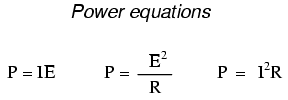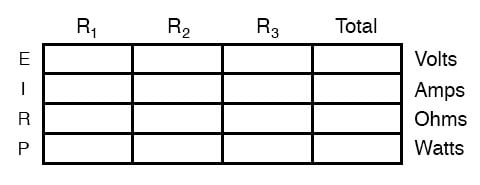# Power Calculations

## Chapter 5 - Series And Parallel Circuits

When calculating the power dissipation of resistive components, use any one of the three power equations to derive the answer from values of voltage, current, and/or resistance pertaining to each component:This is easily managed by adding another row to our familiar table of voltages, currents, and resistances:Power for any particular table column can be found by the appropriate Ohm’s Law equation (appropriate based on what figures are present for E, I, and R in that column).

An interesting rule for total power versus individual power is that it is additive for any configuration of the circuit: series, parallel, series/parallel, or otherwise. Power is a measure of the rate of work, and since power dissipated must equal the total power applied by the source(s) (as per the Law of Conservation of Energy in physics), circuit configuration has no effect on the mathematics.

REVIEW:

• Power is additive in any configuration of resistive circuit: PTotal = P1 + P2 + . . . Pn

View our collection of Power Calculators in our Tools section.

RELATED WORKSHEET:

• Share
Published under the terms and conditions of the Design Science License